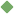factorial 1. [ adjective ] of or relating to factorials To share this definition press "text" (Facebook, Twitter) or "link" (blog, mail) then paste text link 2. [ noun ] (mathematics) the product of all the integers up to and including a given integer Examples: "1, 2, 6, 24, and 120 are factorials" Related terms: product Similar spelling: factor   factor_i   factor_ii   factorize   factorise   factoring   factor_xi   factor_iii   factor_xii   factor_vii   factory   factor_xiii   factor_viii   factor_x   factor_v   factory_farm   factory-made   factor_ix   factor_iv   factor_in   faster   factor_out